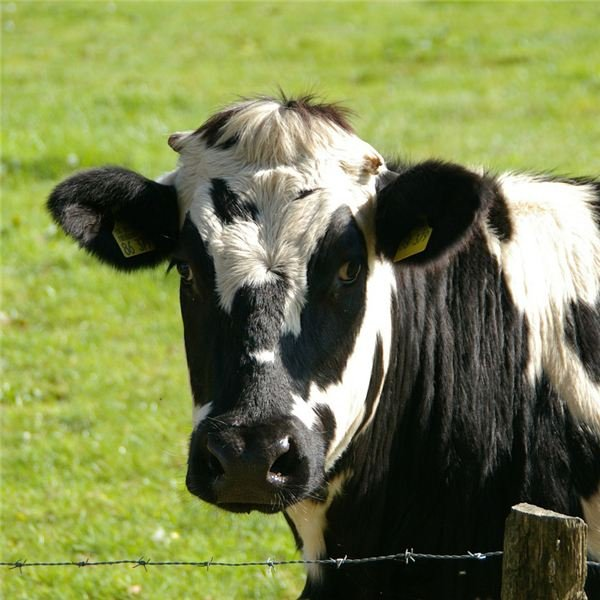Teaching the Basic Facts of Division: 3rd Grade Math Lesson Plan

Lesson Objective: The lesson is aligned to the Common Core State Standards for Mathematics –

3.OA.2 Operations and Algebraic Thinking – Interpret whole-number quotients of whole numbers equally shared.

Materials Required: pictures of cows, spiders, desks in a row, peg boards, and pegs

Lesson Procedure

Start by working through these examples with your class and walking your students through the thought process used to solve these problems.

Cows and EarsJack counts the total number of ears on the cows in the pasture. He says there are 14 ears. Each cow has 2 ears. How many cows are in the pasture? Write the equation that can be used to solve the problem.

Associate the total number of ears and the number of ears on each cow as the total number of objects and the number of objects in each group, and use that information to find the number of groups. (In this case, 1 group = 1 cow.)

Answer: 7 cows, 14 / 2 = 7

Spiders and Legs

Jasper counts the number of legs on the spiders. He counts 24 legs. There are 8 legs on each spider. How many spiders are there? Write the equation that can be used to solve the problem.

Associate the total number of legs and the number of legs on each spider as the total number of objects and the number of objects in each group.

Answer: 3 spiders, 24 / 8 = 3

Individual or Group Work

Have students work on their own or in groups to solve the following problems.

1. Bicycle and Wheels – There are 8 bicycle wheels in the repair shop. Reese wants to build bicycles using the 8 wheels he found in the repair shop. each bicycle will have 2 wheels. How many bicycles can Reese build using the 8 wheels? Write the equation that can be used to solve the problem.

Associate the total number of wheels and the number of wheels on each bicycle as the total number of objects and the number of objects in each group.

2. Peg Board and Pegs – Emily has 35 pegs for her peg board. Each row on the peg board will hold 7 pegs. How many rows will Emily need for her pegs?

Associate the total number of pegs and the number of pegs in each as the total number of objects and the number of objects in each group.

Solve the problems below. Write the equation that can be used to solve each problem.

Each cow has 2 ears.

3. There are 20 ears. How many cows are there?

4. There are 24 ears. How many cows are there?

5. There are 8 ears. How many cows are there?

6. There are 18 ears. How many cows are there?

7. There are 6 ears. How many cows are there?

Each peg board can hold 7 pegs in each row.

8. There are 56 pegs. How many rows are needed?

9. There are 14 pegs. How many rows are needed?

10. There are 21 pegs. How many rows are needed?

11. There are 63 pegs. How many rows are needed?

12. There are 42 pegs. How many rows are needed?

13. There are 28 pegs. How many rows are needed?

14. There are 49 pegs. How many rows are needed?

Each group has 6 objects.

15. There are 12 objects. How many groups are needed?

16. There are 54 objects. How many groups are needed?

17. There are 48 objects. How many groups are needed?

18. There are 60 objects. How many groups are needed?

19. There are 72 objects. How many groups are needed?

20. There are 66 objects. How many groups are needed?

1. 4 bicycles, 8 / 2 = 4

2. 5 rows, 35 / 7 = 5

3. 10 cows, 20 / 2 =10

4. 12 cows, 24 / 2 = 12

5. 4 cows, 8 / 2 = 4

6. 9 cows, 18 / 2 = 9

7. 3 cows, 6 / 2 = 3

8. 8 rows, 56 / 7 = 8

9. 2 rows, 14 / 7 = 2

10. 3 rows, 21 / 7 = 3

11. 9 rows, 63 / 7 = 9

12. 6 rows, 42 / 7 = 6

13. 4 rows, 28 / 7 = 4

14. 7 rows, 49 / 7 = 7

15. 2 groups, 12 / 6 = 2

16. 9 groups, 54 / 6 = 9

17. 8 groups 48 / 6 = 8

18. 10 groups, 60 / 6 = 10

19. 12, 72 / 6 = 12

20. 11 groups, 66 / 6 = 11

Goal: Students should be able to determine the quotient of two whole numbers.

This post is part of the series: Introducing Multiplication and Division in 3rd Grade Math

The activities in these lesson plans are designed to help your students understand the basic facts of multiplication and division, and relate them to problems that involve finding the number of objects in a group.